# Exponential equation - math word problems

#### Number of problems found: 54

• Exponential equationSolve exponential equation (in real numbers): 98x-2=9
• Exponential equationIn the set R solve the equation: ?
• Exponential equationFind x, if 625 ^ x = 5 The equation is exponential because the unknown is in the exponential power of 625
• Exponential equationSolve for x: (4^x):0,5=2/64.
• Exponential equationDetermine the value of having y in the expression (3^y): (4^-1)=36. Unknown y is a natural number greater than zero.
• Logif ?, what is b?
• Exp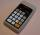If ?, then n is:
• SequenceCalculate what member of the sequence specified by ? has value 86.
• One third power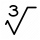Which equation justifies why ten to the one-third power equals the cube root of ten?
• Interest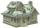What is the annual interest rate on your account if we put 32790 and after 176 days received 33939.2?
• Exponential warmSuppose that a body with temperature T1 is placed in surroundings with temperature T0 different from that of T1. The body will either cool or warm to temperature T(t) after time t, in minutes, where T(t)=T0 + (T1-T0)e^(-kt) . If jello salad with 30 degree
• Coordinate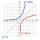Determine missing coordinate of the point M [x, 120] of the graph of the function f bv rule: y = 5x
• Compound interestCalculate time when deposit in the bank with interest 2.5% p.a. doubles.
• Car valueThe car loses value 15% every year. Determine a time (in years) when the price will be halved.
• Geometric sequenceIn the geometric sequence is a4 = 20 a9 = -160. Calculate the first member a1 and quotient q.A radioactive material loses 10% of its mass each year. What proportion will be left there after n=6 years?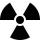After 548 hours decreases the activity of a radioactive substance to 1/9 of the initial value. What is the half-life of the substance?
• What isWhat is the annual percentage increase in the city when the population has tripled in 20 years?
• Geometric progressionIn geometric progression, a1 = 7, q = 5. Find the condition for n to sum first n members is: sn≤217.
• DemographicsThe population grew in the city in 10 years from 30000 to 34000. What is the average annual percentage increase of population?

Do you have an interesting mathematical word problem that you can't solve it? Submit a math problem, and we can try to solve it.

We will send a solution to your e-mail address. Solved examples are also published here. Please enter the e-mail correctly and check whether you don't have a full mailbox.

Please do not submit problems from current active competitions such as Mathematical Olympiad, correspondence seminars etc...